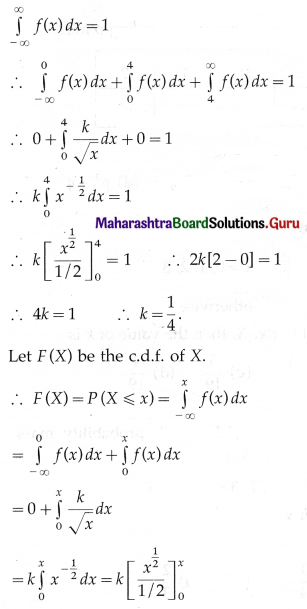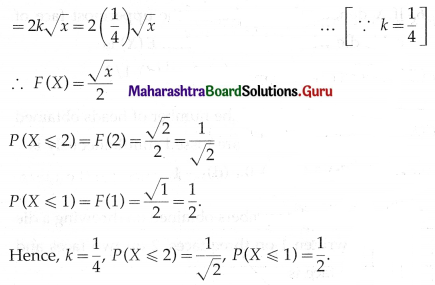Balbharti 12th Maharashtra State Board Maths Solutions Book Pdf Chapter 7 Probability Distributions Miscellaneous Exercise 7 Questions and Answers.

## Maharashtra State Board 12th Maths Solutions Chapter 7 Probability Distributions Miscellaneous Exercise 7

(I) Choose the correct option from the given alternatives:

Question 1.
P.d.f. of a c.r.v. X is f(x) = 6x(1 – x), for 0 ≤ x ≤ 1 and = 0, otherwise (elsewhere) If P(X < a) = P(X > a), then a =
(a) 1
(b) $$\frac{1}{2}$$
(c) $$\frac{1}{3}$$
(d) $$\frac{1}{4}$$
(b) $$\frac{1}{2}$$

Question 2.
If the p.d.f. of a c.r.v. X is f(x) = 3(1 – 2x2), for 0 < x < 1 and = 0, otherwise (elsewhere), then the c.d.f. of X is F(x) =
(a) 2x – 3x2
(b) 3x – 4x3
(c) 3x – 2x3
(d) 2x3 – 3x
(c) 3x – 2x3Question 3.
If the p.d.f. of a c.r.v. X is f(x) = $$\frac{x^{2}}{18}$$, for -3 < x < 3 and = 0, otherwise, then P(|X| < 1) =
(a) $$\frac{1}{27}$$
(b) $$\frac{1}{28}$$
(c) $$\frac{1}{29}$$
(d) $$\frac{1}{26}$$
(a) $$\frac{1}{27}$$

Question 4.
If p.m.f. of a d.r.v. X takes values 0, 1, 2, 3, … which probability P(X = x) = k(x +1) . 5-x, where k is a constant, then P(X = 0) =
(a) $$\frac{7}{25}$$
(b) $$\frac{16}{25}$$
(c) $$\frac{18}{25}$$
(d) $$\frac{19}{25}$$
(b) $$\frac{16}{25}$$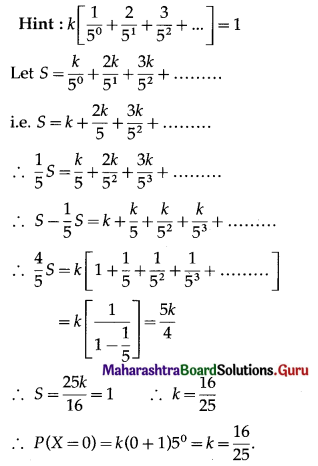Question 5.
If p.m.f. of a d.r.v. X is P(X = x) = $$\frac{\left({ }^{5} \mathrm{C}_{x}\right)}{2^{5}}$$, for x = 0, 1, 2, 3, 4, 5 and = 0, otherwise. If a = P(X ≤ 2) and b = P(X ≥ 3), then
(a) a < b
(b) a > b
(c) a = b
(d) a + b
(c) a = b

Question 6.
If p.m.f. of a d.r.v. X is P(X = x) = $$\frac{x}{n(n+1)}$$, for x = 1, 2, 3, ……, n and = 0, otherwise, then E(X) =
(a) $$\frac{n}{1}+\frac{1}{2}$$
(b) $$\frac{n}{3}+\frac{1}{6}$$
(c) $$\frac{n}{2}+\frac{1}{5}$$
(d) $$\frac{n}{1}+\frac{1}{3}$$
(b) $$\frac{n}{3}+\frac{1}{6}$$Question 7.
If p.m.f. of a d.r.v. X is P(x) = $$\frac{c}{x^{3}}$$, for x = 1, 2, 3 and = 0, otherwise (elsewhere), then E(X) =
(a) $$\frac{343}{297}$$
(b) $$\frac{294}{251}$$
(c) $$\frac{297}{294}$$
(d) $$\frac{294}{297}$$
(b) $$\frac{294}{251}$$

Question 8.
If the d.r.v. X has the following probability distribution: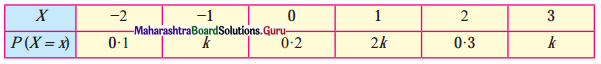then P(X = -1) =
(a) $$\frac{1}{10}$$
(b) $$\frac{2}{10}$$
(c) $$\frac{3}{10}$$
(d) $$\frac{4}{10}$$
(a) $$\frac{1}{10}$$

Question 9.
If the d.r.v. X has the following probability distribution: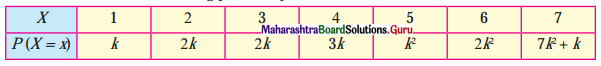then k =
(a) $$\frac{1}{7}$$
(b) $$\frac{1}{8}$$
(c) $$\frac{1}{9}$$
(d) $$\frac{1}{10}$$
(d) $$\frac{1}{10}$$

Question 10.
Find the expected value of X for the following p.m.f.(a) 0.85
(b) -0.35
(c) 0.15
(d) -0.15
(b) -0.35(II) Solve the following:

Question 1.
Identify the random variable as either discrete or continuous in each of the following. If the random variable is discrete, list its possible values:
(i) An economist is interested in the number of unemployed graduates in the town of population 1 lakh.
(ii) Amount of syrup prescribed by a physician.
(iii) The person on a high protein diet is interesting to gain weight in a week.
(iv) 20 white rats are available for an experiment. Twelve rats are males. A scientist randomly selects 5 rats, the number of female rats selected on a specific day.
(v) A highway-safety group is interested in studying the speed (in km/hr) of a car at a checkpoint.
Solution:
(i) Let X = number of unemployed graduates in a town.
Since the population of the town is 1 lakh, X takes the finite values.
∴ random variable X is discrete.
Range = {0, 1, 2, …, 99999, 100000}.

(ii) Let X = amount of syrup prescribed by a physician.
Then X takes uncountable infinite values.
∴ random variable X is continuous.

(iii) Let X = gain of weight in a week
Then X takes uncountable infinite values
∴ random variable X is continuous.

(iv) Let X = number of female rats selected on a specific day.
Since the total number of rats is 20 which includes 12 males and 8 females, X takes the finite values.
∴ random variable X is discrete.
Range = {0, 1, 2, 3, 4, 5}

(v) Let X = speed of .the car in km/hr.
Then X takes uncountable infinite values
∴ random variable X is continuous.

Question 2.
The probability distribution of discrete r.v. X is as follows: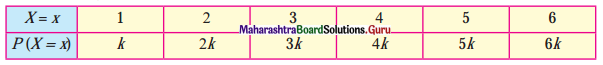(i) Determine the value of k.
(ii) Find P(X ≤ 4), P(2 < X < 4), P(X ≥ 3).
Solution: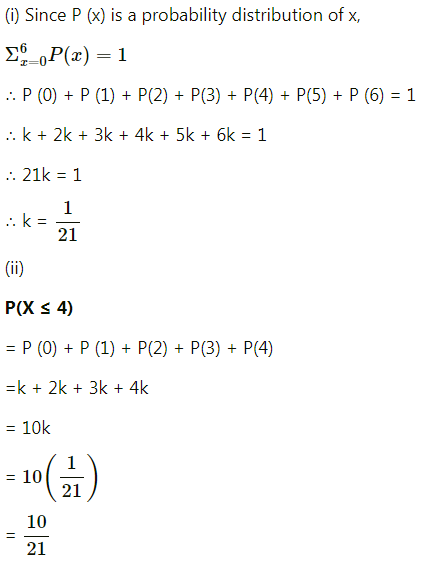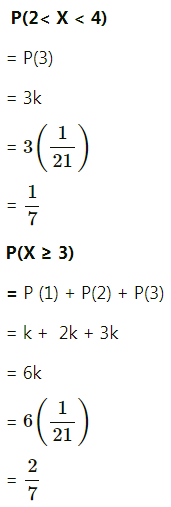Question 3.
The following is the probability distribution of X: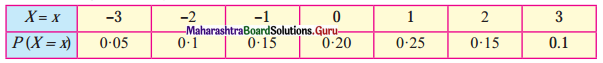Find the probability that
(i) X is positive
(ii) X is non-negative
(iii) X is odd
(iv) X is even.
Solution:
(i) P(X is positive) = P(X = 1) + P(X = 2) + P(X = 3)
= 0.25 + 0.15 + 0.1
= 0.50

(ii) P(X is non-negative)
= P(X = 0) + P(X = 1) + P(X = 2) + P(X = 3)
= 0.20 + 0.25 + 0.15 + 0.1
= 0.70

(iii) P(X is odd)
= P(X = -3) + P(X = -1) + P(X = 1) + P(X = 3)
= 0.05 + 0.15 + 0.25 + 0.1
= 0.55

(iv) P(X is even)
= P(X = -2) + P(X = 0) + P(X = 2)
= 0.10 + 0.20 + 0.15
= 0.45.

Question 4.
The p.m.f. of a r.v. X is given by P(X = x) = x = $$\frac{{ }^{5} \mathrm{C}_{\mathrm{x}}}{2^{5}}$$, for x = 0, 1, 2, 3, 4, 5 and = 0, otherwise. Then show that P(X ≤ 2) = P(X ≥ 3).
Solution:
P(X ≤ 2) = P(X = 0) + P(X = 1) + P(X = 2)
= $$\frac{{ }^{5} \mathrm{C}_{0}}{2^{5}}+\frac{{ }^{5} \mathrm{C}_{1}}{2^{5}}+\frac{{ }^{5} \mathrm{C}_{2}}{2^{5}}$$
= $$\frac{{ }^{5} \mathrm{C}_{5}}{2^{5}}+\frac{{ }^{5} \mathrm{C}_{4}}{2^{5}}+\frac{{ }^{5} \mathrm{C}_{3}}{2^{5}}$$ ………${ }^{n} \mathrm{C}_{r}={ }^{n} \mathrm{C}_{n-r}$
= P(X = 5) + P(X = 4) + P(X = 3)
= P(X ≥ 3)
∴ P(X ≤ 2) = P(X ≥ 3).Question 5.
In the p.m.f. of r.v. X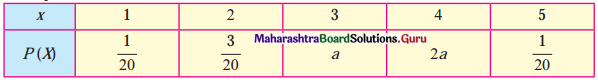Find a and obtain c.d.f. of X.
Solution:
For p.m.f. of a r.v. X
$$\sum_{i=1}^{5} P(X=x)=1$$
∴ P(X = 1) + P(X = 2) + P(X = 3) + P(X = 4) + P(X = 5) = 1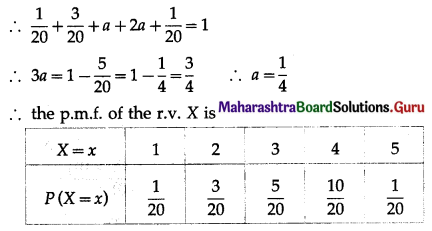Let F(x) be the c.d.f. of X.
Then F(x) = P(X ≤ x)
∴ F(1) = P(X ≤ 1) = P(X = 1) = $$\frac{1}{20}$$
F(2) = P(X ≤ 2) = P(X = 1) + P (X = 2)
$$=\frac{1}{20}+\frac{3}{20}=\frac{4}{20}=\frac{1}{5}$$
P(3) = P(X ≤ 3) = P(X = 1) + P(X = 2) + P(X = 3)
$$=\frac{1}{20}+\frac{3}{20}+\frac{5}{20}=\frac{9}{20}$$
F(4) = P(X ≤ 4) = P(X = 1) + P(X = 2) + P(X = 3) + P(X = 4)
$$=\frac{1}{20}+\frac{3}{20}+\frac{5}{20}+\frac{10}{20}=\frac{19}{20}$$
F(5) = P(X ≤ 5) = P(X = 1) + P(X = 2) + P(X = 3) + P(X = 4) + P(X = 5)
$$=\frac{1}{20}+\frac{3}{20}+\frac{5}{20}+\frac{10}{20}+\frac{1}{20}=\frac{20}{20}=1$$
Hence, the c.d.f. of the random variable X is as follows: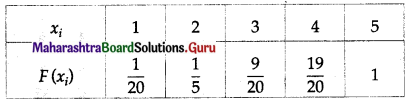Question 6.
A fair coin is tossed 4 times. Let X denote the number of heads obtained. Write down the probability distribution of X. Also, find the formula for p.m.f. of X.
Solution:
When a fair coin is tossed 4 times then the sample space is
S = {HHHH, HHHT, HHTH, HTHH, THHH, HHTT, HTHT, HTTH, THHT, THTH, TTHH, HTTT, THTT, TTHT, TTTH, TTTT}
∴ n(S) = 16
X denotes the number of heads.
∴ X can take the value 0, 1, 2, 3, 4
When X = 0, then X = {TTTT}
∴ n (X) = 1
∴ P(X = 0) = $$\frac{n(X)}{n(S)}=\frac{1}{16}=\frac{{ }^{4} \mathrm{C}_{0}}{16}$$
When X = 1, then
X = {HTTT, THTT, TTHT, TTTH}
∴ n(X) = 4
∴ P(X = 1) = $$\frac{n(X)}{n(S)}=\frac{4}{16}=\frac{{ }^{4} C_{1}}{16}$$
When X = 2, then
X = {HHTT, HTHT, HTTH, THHT, THTH, TTHH}
∴ n(X) = 6
∴ P(X = 2) = $$\frac{n(X)}{n(S)}=\frac{6}{16}=\frac{{ }^{4} \mathrm{C}_{2}}{16}$$
When X = 3, then
X = {HHHT, HHTH, HTHH, THHH}
∴ n(X) = 4
∴ P(X = 3) = $$\frac{n(X)}{n(S)}=\frac{4}{16}=\frac{{ }^{4} C_{3}}{16}$$
When X = 4, then X = {HHHH}
∴ n(X) = 1
∴ P(X = 4) = $$\frac{n(X)}{n(S)}=\frac{1}{16}=\frac{{ }^{4} \mathrm{C}_{4}}{16}$$
∴ the probability distribution of X is as follows: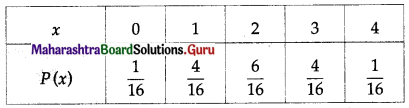Also, the formula for p.m.f. of X is
P(x) = $$\frac{{ }^{4} \mathrm{C}_{x}}{16}$$, x = 0, 1, 2, 3, 4 and = 0, otherwise.Question 7.
Find the probability distribution of the number of successes in two tosses of a die, where success is defined as
(i) number greater than 4
(ii) six appear on at least one die.
Solution:
When a die is tossed two times, we obtain (6 × 6) = 36 number of observations.
Let X be the random variable, which represents the number of successes.
Here, success refers to the number greater than 4.
P(X = 0) = P(number less than or equal to 4 on both the tosses)
= $$\frac{4}{6} \times \frac{4}{6}=\frac{16}{36}=\frac{4}{9}$$
P(X = 1) = P(number less than or equal to 4 on first toss and greater than 4 on second toss) + P(number greater than 4 on first toss and less than or equal to 4 on second toss)
= $$\frac{4}{6} \times \frac{2}{6}+\frac{4}{6} \times \frac{2}{6}$$
= $$\frac{8}{36}+\frac{8}{36}$$
= $$\frac{16}{36}$$
= $$\frac{4}{9}$$
P(X = 2) = P(number greater than 4 on both the tosses)
= $$\frac{2}{6} \times \frac{2}{6}=\frac{4}{36}=\frac{1}{9}$$
Thus, the probability distribution is as follows: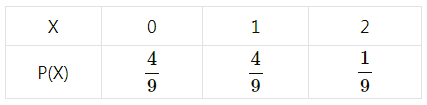(ii) Here, success means six appears on at least one die.
P(Y = 0) = P(six appears on none of the dice) = $$\frac{5}{6} \times \frac{5}{6}=\frac{25}{36}$$
P(Y = 1) = P(six appears on none of the dice x six appears on at least one of the dice ) + P(six appears on none of the dice x six appears on at least one of the dice)
= $$\frac{1}{6} \times \frac{5}{6}+\frac{1}{6} \times \frac{5}{6}=\frac{5}{36}+\frac{5}{36}=\frac{10}{36}$$
P(Y = 2) = P(six appears on at least one of the dice) = $$\frac{1}{6} \times \frac{1}{6}=\frac{1}{36}$$
Thus, the required probability distribution is as follows: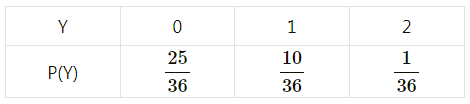Question 8.
A random variable X has the following probability distribution: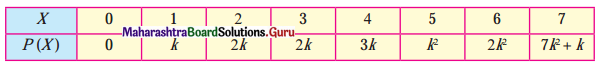Determine:
(i) k
(ii) P(X > 6)
(iii) P(0 < X < 3).Question 9.
The following is the c.d.f. of a r.v. X: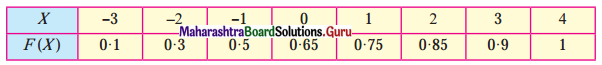Find
(i) p.m.f. of X
(ii) P( -1 ≤ X ≤ 2)
(iii) P(X ≤ X > 0).
Solution:
(i) From the given table
F(-3) = 0.1, F(-2) = 0.3, F(-1) = 0.5
F(0) = 0.65, f(1) = 0.75, F(2) = 0.85
F(3) = 0.9, F(4) = 1
P(X = -3) = F(-3) = 0.1
P(X = -2) = F(-2) – F(-3) = 0.3 – 0.1 = 0.2
P(X = -1) = F(-1) – F(-2) = 0.5 – 0.3 = 0.2
P(X = 0) = F(0) – F(-1) = 0.65 – 0.5 = 0.15
P(X = 1) = F(1) – F(0) = 0.75 – 0.65 = 0.1
P(X = 2) = F(2) – F(1) = 0.85 – 0.75 = 0.1
P(X = 3) = F(3) – F(2) = 0.9 – 0.85 = 0.1
P(X = 4) = F(4) – F(3) = 1 – 0.9 = 0.1
∴ the p.m.f of X is as follows: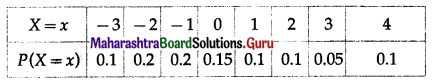(ii) P(-1 ≤ X ≤ 2) = P(X = -1) + P(X = 0) + P(X = 1) + P(X = 2)
= 0.2 + 0.15 + 0.1 + 0.1
= 0.55

(iii) (X ≤ 3) ∩ (X > 0)
= { -3, -2, -1, 0, 1, 2, 3} n {1, 2, 3, 4}
= {1, 2, 3}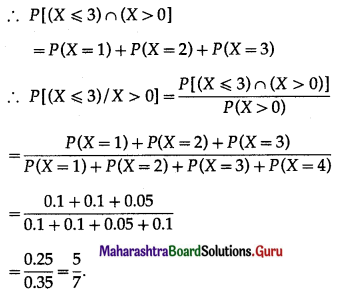Question 10.
Find the expected value, variance, and standard deviation of the random variable whose p.m.f’s are given below: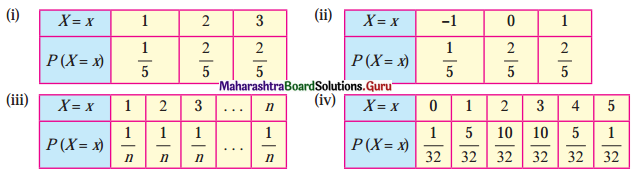Solution:
(i) We construct the following table to find the expected value, variance, and standard deviation: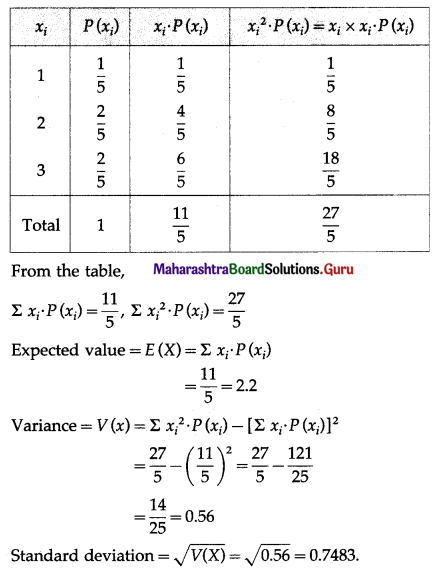(ii) We construct the following table to find the expected value, variance, and standard deviation: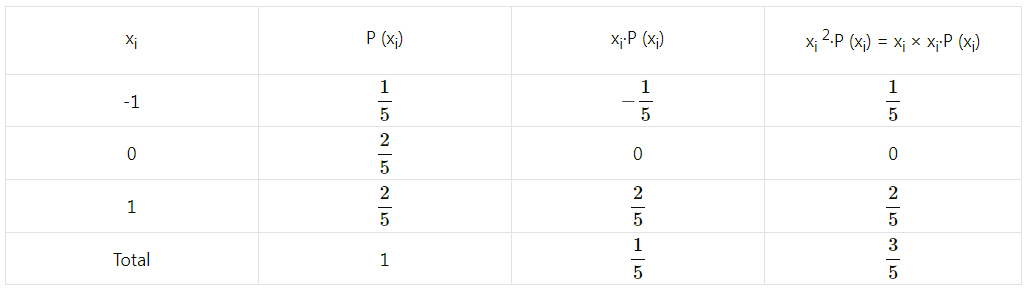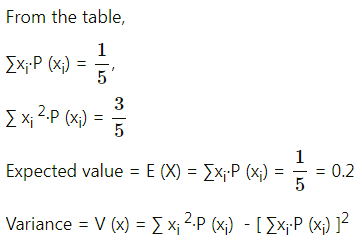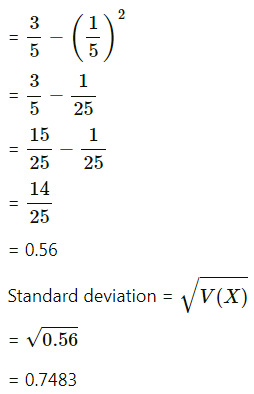(iii) We construct the following table to find the expected value, variance, and standard deviation: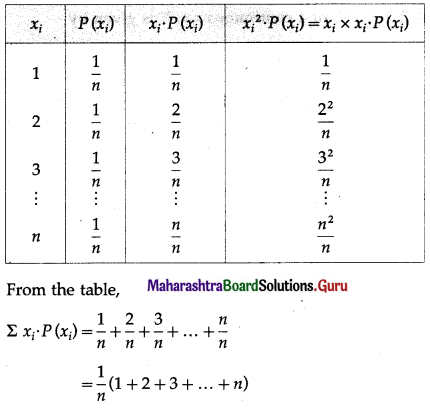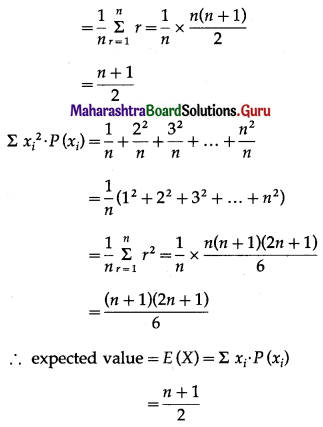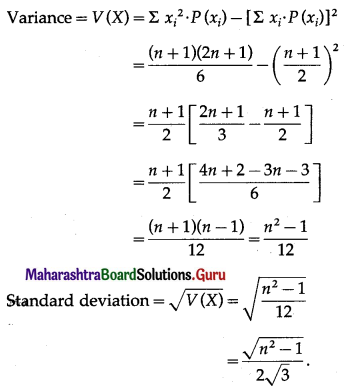(iv) We construct the following table to find the expected value, variance, and standard deviation: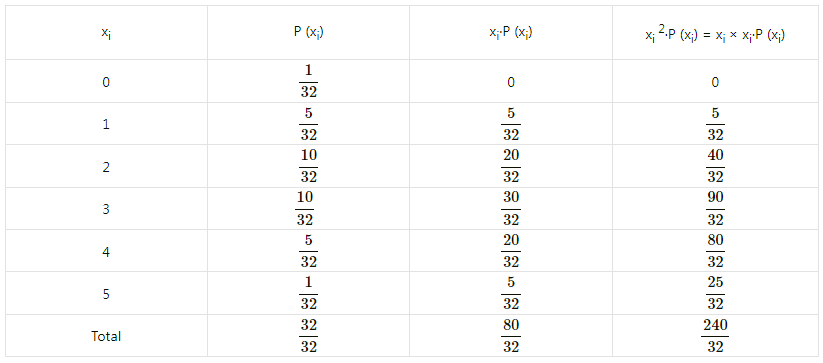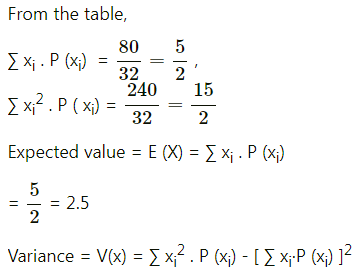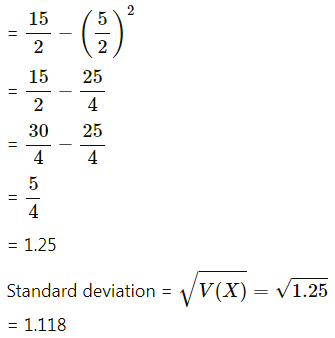Question 11.
A player tosses two wins. He wins ₹ 10 if 2 heads appear, ₹ 5 if 1 head appears and ₹ 2 if no head appears. Find the expected winning amount and variance of the winning amount.
Solution:
When a coin is tossed twice, the sample space is
S = {HH, HT, TH, HH}
Let X denote the amount he wins.
Then X takes values 10, 5, 2.
P(X = 10) = P(2 heads appear) = $$\frac{1}{4}$$
P(X = 5) = P(1 head appears) = $$\frac{2}{4}$$ = $$\frac{1}{2}$$
P(X = 2) = P(no head appears) = $$\frac{1}{4}$$
We construct the following table to calculate the mean and the variance of X: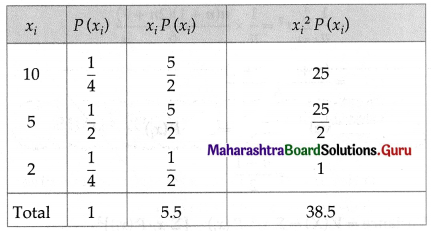From the table Σxi . P(xi) = 5.5, $$\Sigma x_{i}^{2} \cdot P\left(x_{i}\right)$$ = 38.5
E(X) = Σxi . P(xi) = 5.5
Var(X) = $$\Sigma x_{i}^{2} \cdot P\left(x_{i}\right)$$ – [E(X)]2
= 38.5 – (5.5)2
= 38.5 – 30.25
= 8.25
∴ Hence, expected winning amount = ₹ 5.5 and variance of winning amount = ₹ 8.25.

Question 12.
Let the p.m.f. of r.v. X be P(x) = $$\frac{3-x}{10}$$, for x = -1, 0, 1, 2 and = 0, otherwise.
Calculate E(X) and Var(X).
Solution:
P(X) = $$\frac{3-x}{10}$$
X takes values -1, 0, 1, 2
P(X = -1) = P(-1) = $$\frac{3+1}{10}=\frac{4}{10}$$
P(X = 0) = P(0) = $$\frac{3-0}{10}=\frac{3}{10}$$
P(X = 1) = P(1) = $$\frac{3-1}{10}=\frac{2}{10}$$
P(X = 2) = P(2) = $$\frac{3-2}{10}=\frac{1}{10}$$
We construct the following table to calculate the mean and variance of X: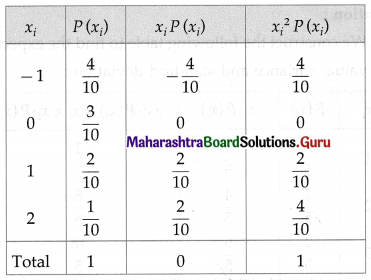From the table
ΣxiP(xi) = 0 and $$\Sigma x_{i}{ }^{2} \cdot P\left(x_{i}\right)$$ = 1
E(X) = ΣxiP(xi) = 0
Var(X) = $$\Sigma x_{i}{ }^{2} \cdot P\left(x_{i}\right)$$ – [E(X)]2
= 1 – 0
= 1
Hence, E(X) = 0, Var (X) = 1.Question 13.
Suppose the error involved in making a certain measurement is a continuous r.v. X with p.d.f.
f(x) = k(4 – x2), -2 ≤ x ≤ 2 and = 0 otherwise.
Compute
(i) P(X > 0)
(ii) P(-1 < X < 1)
(iii) P(X < -0.5 or X > 0.5).
Solution:
(i) P(X > 0)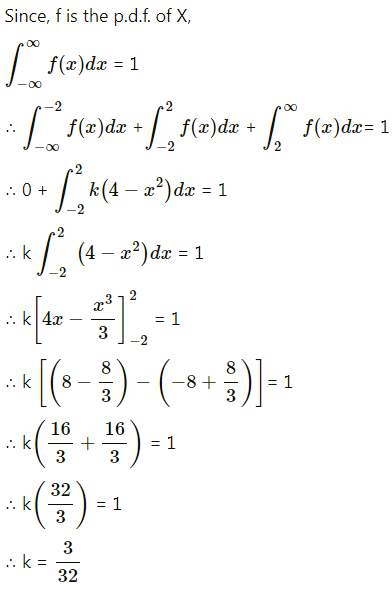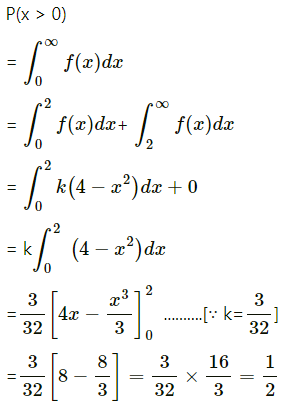(ii) P(-1 < X < 1)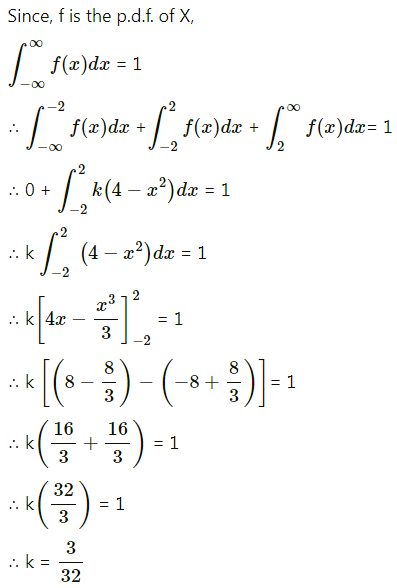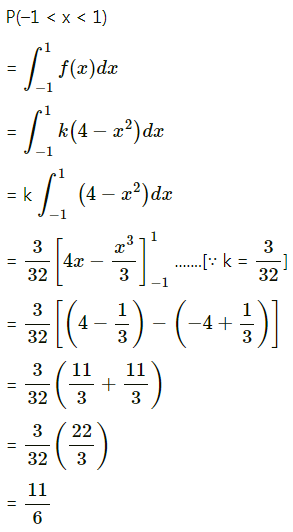(iii) P(X < -0.5 or X > 0.5)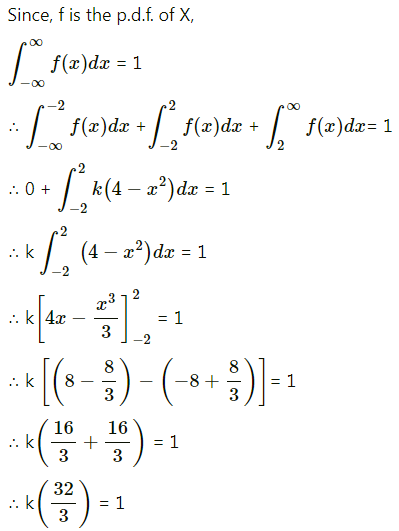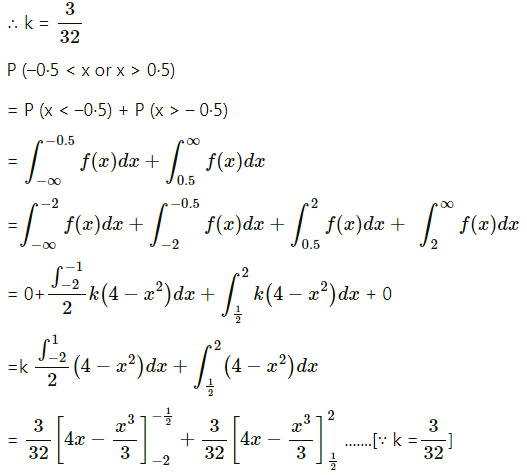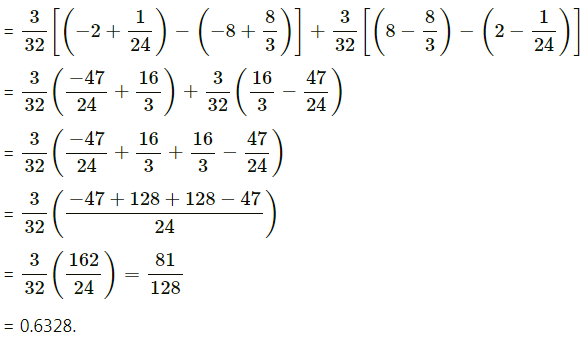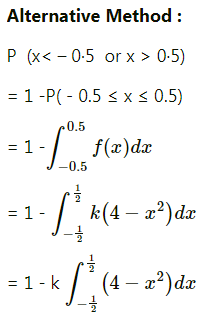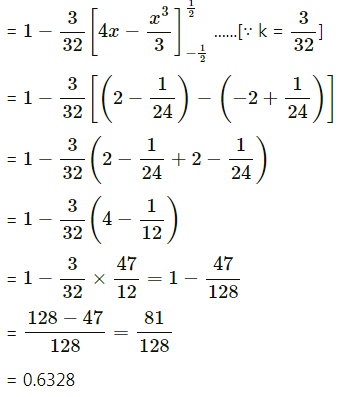Question 14.
The p.d.f. of a continuous r.v. X is given by f(x) = $$\frac{1}{2 a}$$, for 0 < x < 2a and = 0, otherwise. Show that P( X < $$\frac{a}{2}$$) = P(X > $$\frac{3a}{2}$$)
Solution: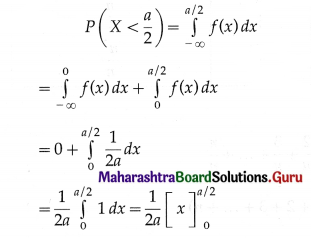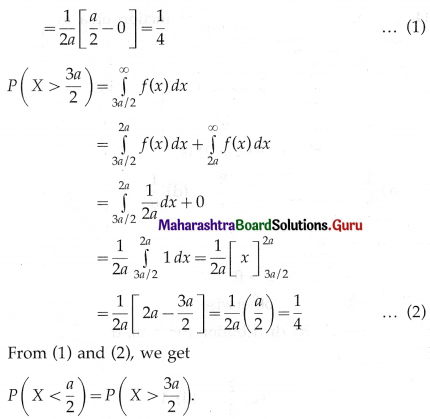Question 15.
The p.d.f. of r.v. X is given by f(x) = $$\frac{k}{\sqrt{x}}$$, for 0 < x < 4 and = 0, otherwise. Determine k. Determine c.d.f. of X and hence find P(X ≤ 2) and P(X ≤ 1).
Solution:
Since f is p.d.f. of the r.v. X,